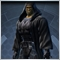# Need MA cross assistance for my EA36

Hello,

Can someone help me with my EA that I am building?  There is one piece that I am unable to understand.  Currently my EA opens the trade as soon as my EMAs cross.  But I only want the trade to open if the EMAs are confirmed at the candle close, along with my other criteria.  I've seen others use arrays, but I am having trouble incorporating the logic into my EA code.

Here are my Criteria:

BUY:

10EMA crossed over the 3EMA; MFI >= 50; RSI >= 50; Momentum >= 50; MACD (5, 10, 1) Signal line is >= 0; Stoch (10, 50, 3) Signal >= Baseline; Open on next bar

Close Buy when MFI or RSI cross below the 50

SELL:

10EMA crossed below the 3EMA; MFI <= 50; RSI <= 50; Momentum <= 50; MACD (5, 10, 1) Signal line is <= 0; Stoch (10, 50, 3) Signal <= Baseline; Open on next bar

Close Sell when MFI or RSI cross above the 50

I have attached my mq4 file

Thanks!

Files:151

```if(isNewBar //Send order when new bar opens
&& Cross(4, iMA(NULL, PERIOD_CURRENT, SlowEMA, 0, MODE_EMA, PRICE_CLOSE, 1) > iMA(NULL, PERIOD_CURRENT, FastEMA, 0, MODE_EMA, PRICE_CLOSE, 1)) //Moving Average crosses above Moving Average
&& iRSI(NULL, PERIOD_CURRENT, RSI, PRICE_CLOSE, 1) >= 50 //Relative Strength Index >= fixed value
&& iMFI(NULL, PERIOD_CURRENT, MFI, 1) >= 50 //Money Flow Index >= fixed value
&& iMomentum(NULL, PERIOD_CURRENT, Momentum, PRICE_CLOSE, 1) >= 50 //Momentum >= fixed value
&& iMACD(NULL, PERIOD_CURRENT, MACD_Fast, MACD_Slow, MACD_SMA, PRICE_CLOSE, MODE_MAIN, 1) >= 0 //MACD >= fixed value
&& iStochastic(NULL, PERIOD_CURRENT, 10, 50, 3, MODE_SMA, 0, MODE_SIGNAL, 1) >= iStochastic(NULL, PERIOD_CURRENT, 10, 50, 3, MODE_SMA, 0, MODE_MAIN, 1) //Stochastic Oscillator >= Stochastic Oscillator
)```
```if(isNewBar //Send order when new bar opens
&& Cross(5, iMA(NULL, PERIOD_CURRENT, SlowEMA, 0, MODE_EMA, PRICE_CLOSE, 1) < iMA(NULL, PERIOD_CURRENT, FastEMA, 0, MODE_EMA, PRICE_CLOSE, 1)) //Moving Average crosses below Moving Average
&& iRSI(NULL, PERIOD_CURRENT, RSI, PRICE_CLOSE, 1) <= 50 //Relative Strength Index <= fixed value
&& iMFI(NULL, PERIOD_CURRENT, MFI, 1) <= 50 //Money Flow Index <= fixed value
&& iMomentum(NULL, PERIOD_CURRENT, Momentum, PRICE_CLOSE, 1) <= 50 //Momentum <= fixed value
&& iMACD(NULL, PERIOD_CURRENT, MACD_Fast, MACD_Slow, MACD_SMA, PRICE_CLOSE, MODE_MAIN, 1) <= 0 //MACD <= fixed value
&& iStochastic(NULL, PERIOD_CURRENT, 10, 50, 3, MODE_SMA, 0, MODE_SIGNAL, 1) <= iStochastic(NULL, PERIOD_CURRENT, 10, 50, 3, MODE_SMA, 0, MODE_MAIN, 1) //Stochastic Oscillator <= Stochastic Oscillator
)```

That might work... but your code seems unnecessarily overcomplicated. Try this Cross function

```int Cross(){
10EMA_2=iMA(Symbol(),0,10,0,MODE_EMA,PRICE_CLOSE,2);
10EMA_1=iMA(Symbol(),0,10,0,MODE_EMA,PRICE_CLOSE,1);
3EMA_2=iMA(Symbol(),0,3,0,MODE_EMA,PRICE_CLOSE,2);
3EMA_1=iMA(Symbol(),0,3,0,MODE_EMA,PRICE_CLOSE,1);

if(3EMA_2<=10EMA_2 && 3EMA_1>10EMA_1){return(1);}
if(3EMA_2>=10EMA_2 && 3EMA_1<10EMA_1){return(-1);}
return(0);
}

if(Cross()>0 && RSI && Momentum && MACD && ...){OpenBuyOrder...}
if(Cross()<0 && RSI && Momentum && MACD && ...){OpenSellOrder...}```

And the NewBar function is weird..

```bool NewBar()
{
static datetime LastTime = 0;
bool ret = Time > LastTime && LastTime > 0;
LastTime = Time;
return(ret);
}

Huh?

bool NewBar(){
if(bars!=Bars){//new bar
bars=Bars;//reset bars to current bar value
return(true);
}
else return(false);
}```36

Chad Magruder:

That might work... but your code seems unnecessarily overcomplicated. Try this Cross function

And the NewBar function is weird..

Thanks @Chad Magruder I will give this a try tonite!36

addown:

Thanks @Chad Magruder I will give this a try tonite

Chad Magruder:

That might work... but your code seems unnecessarily overcomplicated. Try this Cross function

And the NewBar function is weird..

The compiler is complaining about the operator missing from 10EMA_1; 10EMA_2; 3EMA_1; and 3EMA_2 in the two IF statements with the returns.  I'm not really what the operator should be for these.22

Try Renaming the Variable names to begin with a Character not a number.41

Hello Addown...

I'am looking for a code wich does exactly what you dont want your EA to do. I need mine to open a position as soon as the MAs crosses eachother...
could you share this specific code, please?

I would really, really appreciate that cuz i've been looking for something like that for a while.

Thanks in advance